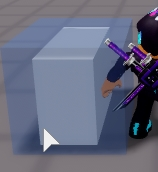# How would I go about making a part snap to a grid

Basically, I am working on a Sandbox tycoon and it’s mainly gone well. I just can’t seem to figure out how to fix this.This bothers me as I can’t seem to find a good way to snap it on right. I have tried many solutions such as ask others for a direction to go in and they can’t seem to help. If anyone can help me find a possible solution that would be great. All I need is a direction to go in. I don’t need code.

1 Like

This is a small snippet of the tutorial that explains how to snap the part to a grid

Everything looks good, but sometimes we might want to be locked to a grid. To do this we return to our `:CalcPlacementCFrame()` method and round the `x` and `y` variables to the nearest grid value.

``````function Placement:CalcPlacementCFrame(model, position, rotation)
-- one of the properties I didn't explain earlier
local g = self.GridUnit
if (g > 0) then
x = math.sign(x)*((math.abs(x) - math.abs(x) % g) + (size2.x % g))
y = math.sign(y)*((math.abs(y) - math.abs(y) % g) + (size2.y % g))
end
end
``````

Now say we set `GridUnit` to `2` then our placement system will be locked to a 2x2 grid!

1 Like

Thanks! This is exactly what I needed! I will get to work on getting the system fixed.

1 Like